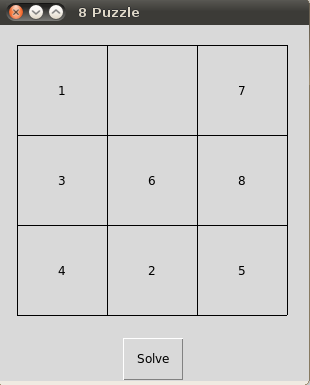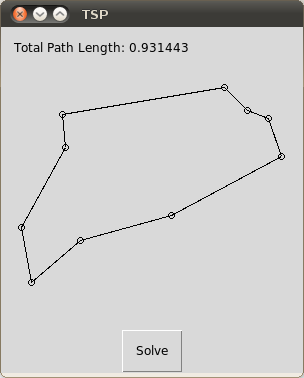Continuing from my last post, I have been dealing with the 4th chapter in AIAMA book which is on informed search methods. Informed search methods use heuristic functions to guide them to goal states quicker so Search.SearcProblem class takes a list of heuristic functions for the problem and in order to use informed search methods you need to provide at least one heuristic function. Heuristic functions provided are assumed to be admissible since this is necessary for some informed search methods to produce optimal solutions. If multiple heuristic functions are given, dominating one (which yields a bigger heuristic value) is used for each node. In order to ensure monotonicity, if a child node’s total estimated path cost (sum of actual path cost so far and heuristic value for that node) is less than its parent’s, its parent’s total path cost is used which is called pathmax equation. I have added the following search methods to the Search module I have implemented.

• Greedy Search: This method only uses the heuristic function to guide the search procedure.
• A* Search: A* uses total estimated path cost (sum of path cost so far and heuristic value) to choose the next node to expand. This simple search method is both complete and optimal making it one of the most important methods.
• Iterative Deepening A* Search: Like the counterpart in uninformed methods, IDA* runs multiple consecutive A* methods each covering more of the search space. However, A* uses a limit on the total estimated path cost not the search tree depth as it was in IDS.

For testing the code and demonstration, I have added heuristic functions for the two problems that I have implemented earlier and solved them with informed methods. I have also included very primitive GUIs for these problems where a random problem is drawn onto window and can be solved with the Solve button.

Misplaced tiles and Manhattan Distance heuristics are implemented for 8Puzzle problem. A sample code and screenshot of GUI can be seen below.Note that some of the randomly generated puzzled are inherently hard and can take a long time to solve it. But worse is that some of the puzzles cannot be solved at all but program will try to find a solution and will probably crash. Take a look at the article on N-Puzzle in Wikipedia for a detailed analysis of this puzzle. The other search problem you can find in source code is yet another famous problem, Traveling Salesman Problem (TSP). I have implemented minimum spanning tree construction with Prim’s algorithm and used the total cost of tree as a heuristic value for TSP. Below you can see the sample code and screenshot.As usual, all source code is implemented with Python 3.1 and I have used Tk for GUI programming.
I’d be happy to hear your questions, comments & suggestions.
Source Code